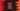# Python string rsplit method explanation with example## Python string rsplit() method:

The rsplit method of python string is used to split a string. It splits the string in words and returns a list with those words. It takes two parameters and both of these optional.

In this post, we will learn how to use this method with examples.

### Definition of rsplit:

The rsplit method is defined as like below:

``str.rsplit([separator, [maxSplit]])``

It takes two parameters:

• separator is the separator to used in split. It is an optional value. By default, it takes a space as the separator.
• maxSplit is also an optional value. This is the maximum number of splits to do. By default, it is -1, i.e. it splits all.

### Return value of rsplit:

The rsplit method returns a list of splitted strings.

### Example of rsplit:

Let’s take an example of rsplit:

``````given_str = 'Hello World !!'
result = given_str.rsplit()

print('result: ', result)``````

Here, rsplit is used with given_str. We are not passing any parameter to this method. So, by default it will split the string in space and return one list with all words.

It will print the below output:

``result:  ['Hello', 'World', '!!']``

### Example of rsplit with a given separator:

In the below example, we are passing a separator, _ to rsplit:

``````given_str = 'Hello_World_!!_Hello_Universe'
result = given_str.rsplit('_')

print('result: ', result)``````

It will print:

``result:  ['Hello', 'World', '!!', 'Hello', 'Universe']``

We can use any string as the separator. For example, the below program uses the string ‘and’ as the separator:

``````given_str = 'HelloandWorldand!!andHelloandUniverse'
result = given_str.rsplit('and')

print('result: ', result)``````

It will give the same result:

``result:  ['Hello', 'World', '!!', 'Hello', 'Universe']``

### Example of rsplit with separator and maxSplit:

As I explained above, maxSplit defines the maximum number of splits that can be done. By default, it is -1 and it is an optional value. At max, the output list can have maxSplit + 1 number of elements. Let’s try it with one example:

``````given_str = 'Hello_World_!!_Hello_Universe'
result = given_str.rsplit('_', 2)

print('result: ', result)``````

The value of maxSplit is 2 and thus the output list will include only three items.

``result:  ['Hello_World_!!', 'Hello', 'Universe']``

To split all the words, we will have to pass 4 as the maxSplit:

``````given_str = 'Hello_World_!!_Hello_Universe'
result = given_str.rsplit('_', 4)

print('result: ', result)``````

It will print:

``result:  ['Hello', 'World', '!!', 'Hello', 'Universe']``

If we pass anything greater than 4, it will always return the same list.

e.g.:

``````given_str = 'Hello_World_!!_Hello_Universe'
result = given_str.rsplit('_', 40)

print('result: ', result)``````

This will print:

``result:  ['Hello', 'World', '!!', 'Hello', 'Universe']``

### Difference between split and rsplit in Python:

Both split and rsplit methods are used to split a string in Python. Both are similar methods and takes the same parameters. The only difference between split and rsplit is that split starts the splitting from left to right and rsplit from right to left.

To understand the difference, let’s take a look at the below program:

``````given_str = 'Hello_World_!!_Hello_Universe'
result_rsplit = given_str.rsplit('_', 1)
result_split = given_str.split('_', 1)

print('result_rsplit: ', result_rsplit)
print('result_split: ', result_split)``````

This program uses both split and rsplit methods. The maxSplit value is passed as 1 for both. But, since split starts from left and rsplit starts from right, both will print different results.

``````result_rsplit:  ['Hello_World_!!_Hello', 'Universe']
result_split:  ['Hello', 'World_!!_Hello_Universe']``````

### ValueError in rsplit:

If we pass an empty string, it will throw a ValueError. For example,

``````given_str = 'Hello_World_!!_Hello_Universe'
result = given_str.rsplit('')

print('result: ', result)``````

It will throw:

``````Traceback (most recent call last):
File "example.py", line 2, in <module>
result = given_str.rsplit('')
ValueError: empty separator``````

You can use a try-except block

``````given_str = 'Hello_World_!!_Hello_Universe'

try:
result = given_str.rsplit('')
print('result: ', result)
except ValueError:
print('Invalid separator')``````